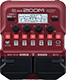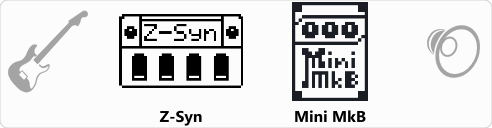# Frog

Discussion in 'Zoom B1/B1X Four' started by Erwin Zettlmeier, Dec 26, 2020.

1. FrogDevice: Zoom B1 Four
Firmware: 2.00

Name on device: Frog
Optimized for: Phones/Speaker

Effects chain:Frog

Effect: "Z-Syn" (Sfx), active - "yes"
"FREQ" = 2
"Range" = 8
"Decay" = 72
"RESO" = 18
"Wave" = 0
"Tone" = 7
"BAL" = 80
"VOL" = 61
"Frequency" = 0
"Range" = 0
"Decay" = 0
"Resonance" = 0
"Wave" = SAW
"Tone" = 0
"Balance" = 0
"Volume" = 0

Effect: "Mini MkB" (Amp simulator), active - "yes"
"Gain" = 50
"VNTG" = 42
"Shape" = 58
"Volume" = 43

Patch Volume: 100